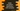# Kotlin groupByTo method explanation with examples## Introduction :

groupByTo method is used to group elements of an array by a key returned by a key selector function. It is similar to groupBy. The only difference is that we can put the result in a mutable map.

In this post, I will show you how it works with different examples.

## Definition :

This method is defined as below :

``groupByTo(destinationMap, keySelector, valueTransform): Map``

Here, keySelector - This function is applied to each element to generate the key valueTransform - applies to each element to change its value destinationMap - Puts the final result in this destination map.

### Example 1:

Let’s take a look at the example below :

``````fun main() {
val strArray = arrayOf("one", "two", "three", "four", "five")

val strMutableList = HashMap<Int, MutableList<String>>()
strArray.groupByTo(strMutableList, { it.length })

print(strMutableList)
}``````

Here, we have one array of strings. strMutableList is a HashMap. We are using groupByTo to group the array elements by its length and we are putting the result the list strMutableList.

It will give the below output :

``{3=[one, two], 4=[four, five], 5=[three]}``

### Example 2:

Let’s take a look at the below example :

``````fun main() {
val playerGroup = listOf("Alex" to "Group-1", "Bob" to "Group-2", "Chandler" to "Group-2", "Albert" to "Group-1")

val playerGroupMap = playerGroup.groupByTo(HashMap(), {it.second} , {it.first})

print(playerGroupMap)
}``````

We are using one list in this example. It uses the group names as the key selector function and the name of player as the value transform function. It groups the players of the same group in a HashMap like below :

``{Group-2=[Bob, Chandler], Group-1=[Alex, Albert]}``

We can create one mutable or immutable HashMap using this method.